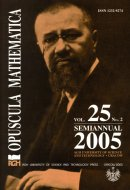Opuscula Math. 25, no. 2 (2005), 307-318

Opuscula Mathematica

# Monotone iteration for infinite systems of parabolic equations

Anna Pudełko

Abstract. In the paper the Cauchy problem for an infinite system of parabolic type equations is studied. The general operators of the parabolic type of second order with variable coefficients are considered and the system is weakly coupled. Among the obtained results there is a theorem on differential inequality as well as the existence and uniqueness theorem in the class of continuous-bounded functions obtained by monotone iterative method.

Keywords: infinite systems, parabolic equations, Cauchy problem, monotone iteration method, differential inequality.

Mathematics Subject Classification: 35K15, 35K55, 35R45.

Full text (pdf)

• Anna Pudełko
• AGH University of Science and Technology, Faculty of Applied Mathematics, al. Mickiewicza 30, 30-059 Kraków, Poland